# For the beam and loading shown, (a) draw the shear and bending-moment diagrams, (b) determine the...

For the beam and loading shown, (a) draw the shear and bending-moment diagrams, (b) determine the maximum absolute values of the shear and bending moment.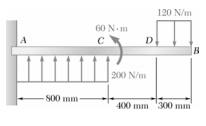120 N/m 60 Nm с A D B 200 N/m 500 mm 400 mm 300 mm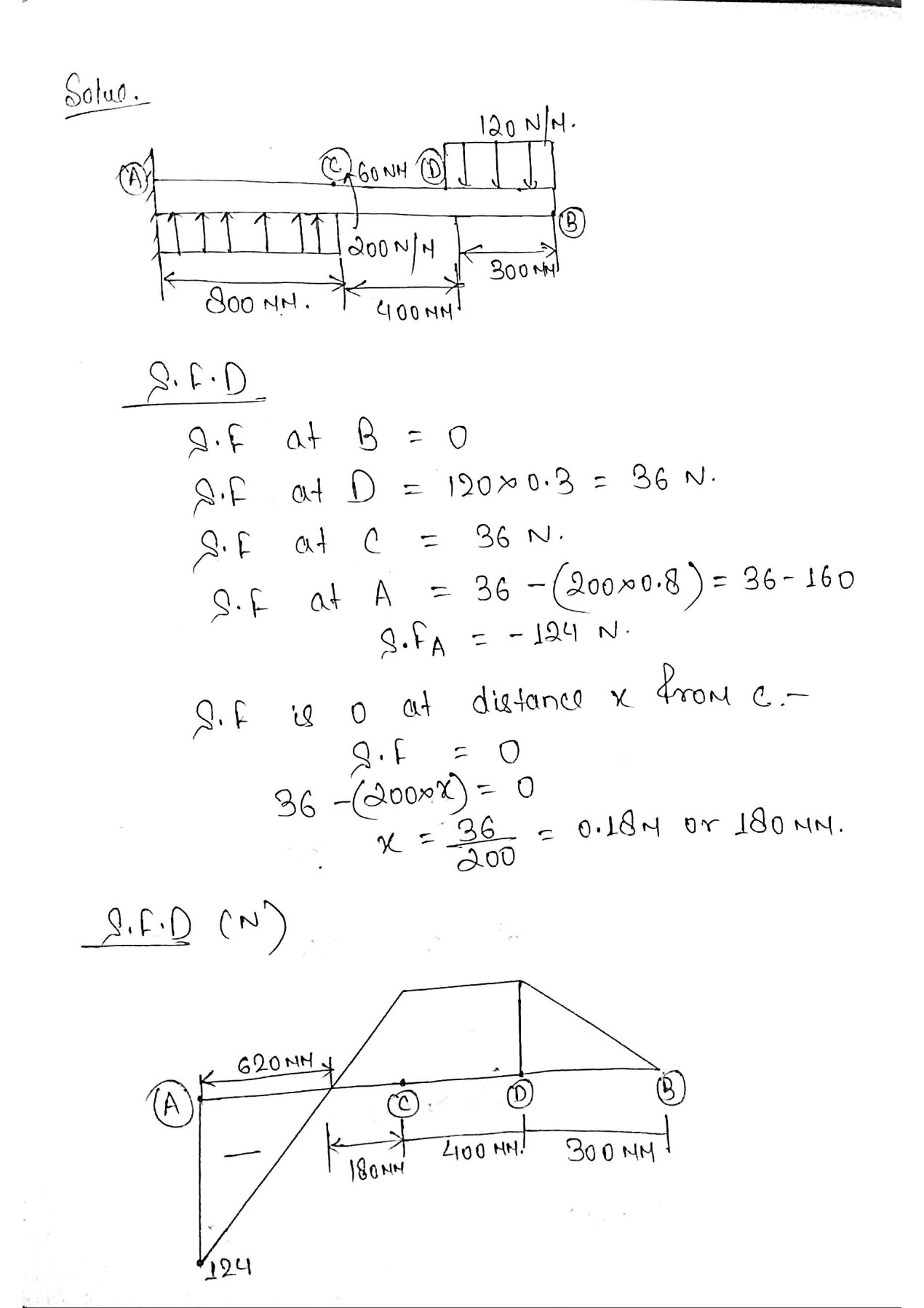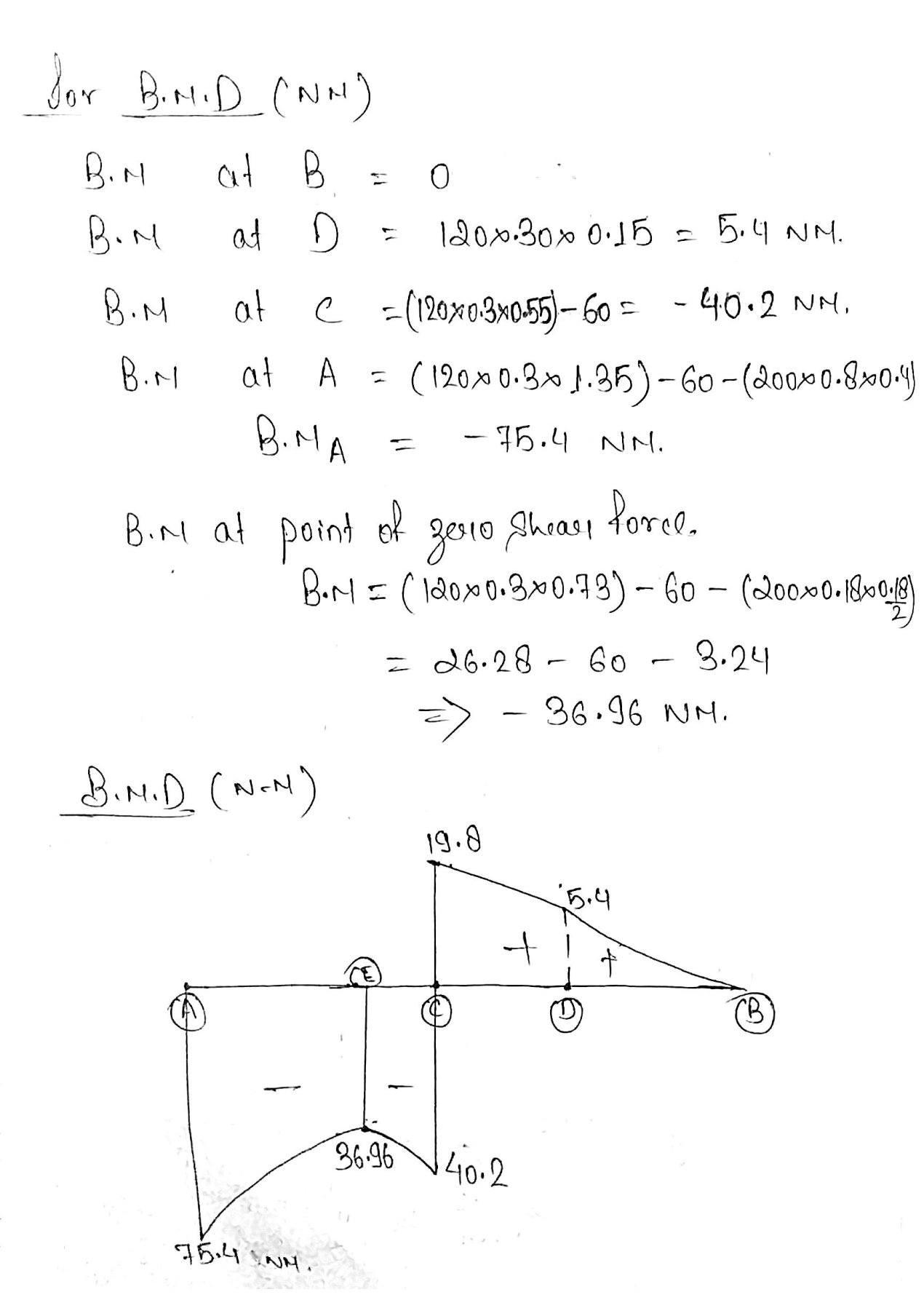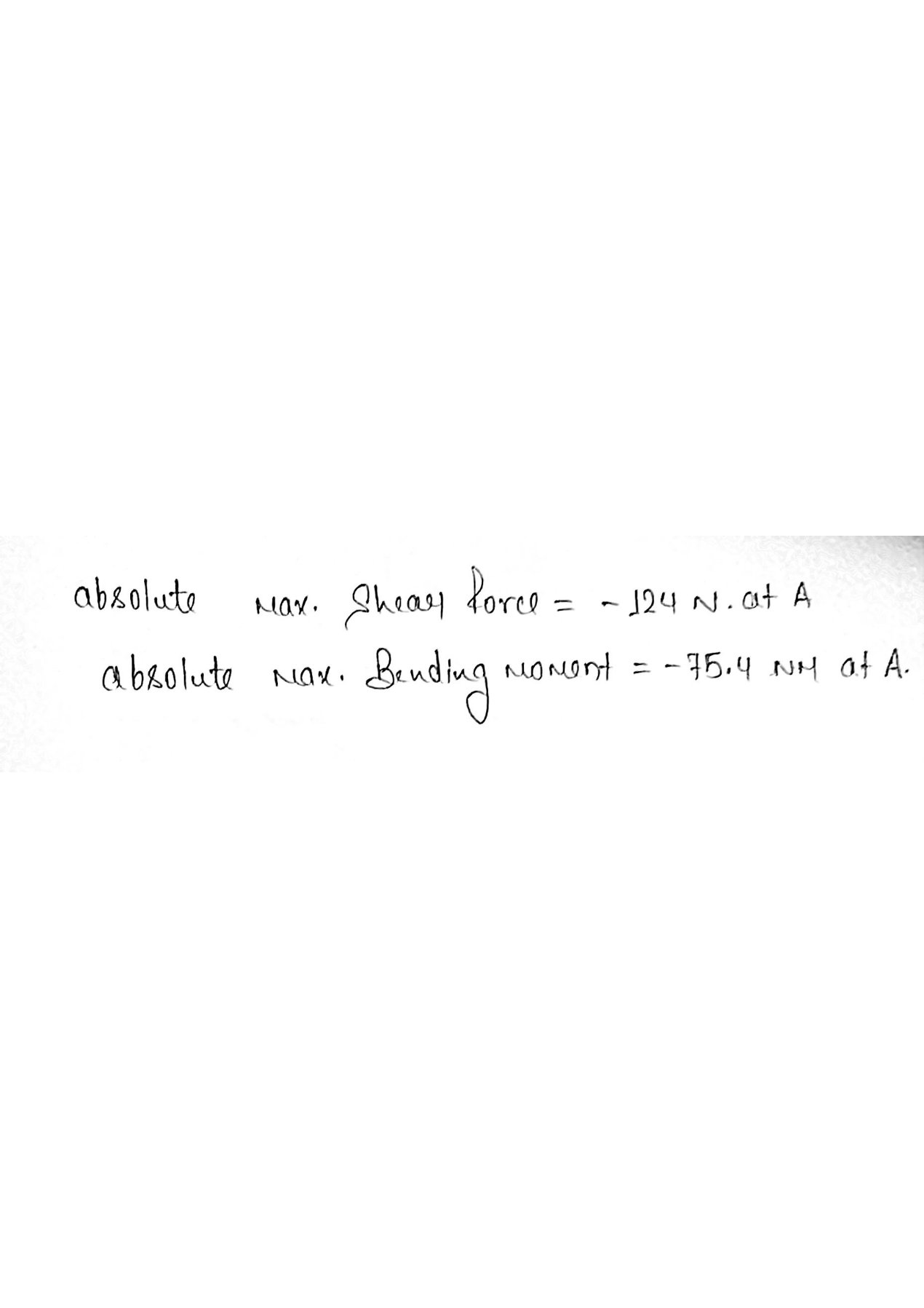#### Earn Coin

Coins can be redeemed for fabulous gifts.

Similar Homework Help Questions
• ### PROBLEM 6 For the beam and loading shown, (a) draw the shear and bending-moment diagrams, (b)...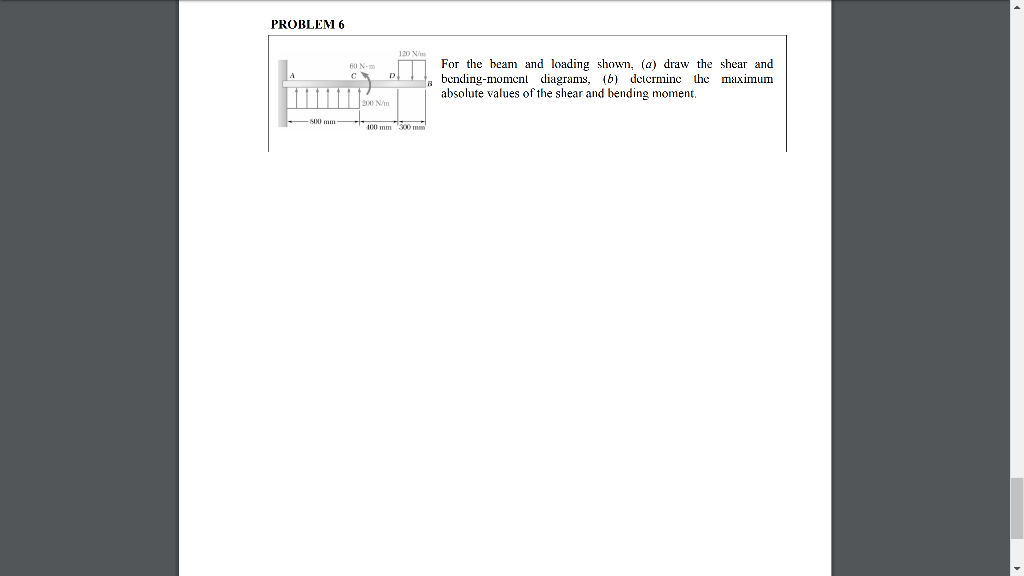PROBLEM 6 For the beam and loading shown, (a) draw the shear and bending-moment diagrams, (b) determine the maximum absolute values of the shear and bending moment. PROBLEM 6 120 W BON For the beam and loading shown, (a) draw the shear and bending-moment diagrams, (6) detcrminc the maximum absolute values of the shear and bending moment, 90 mm 100 mm

• ### For the beam and loading shown draw the shear and bending moment diagrams and then find...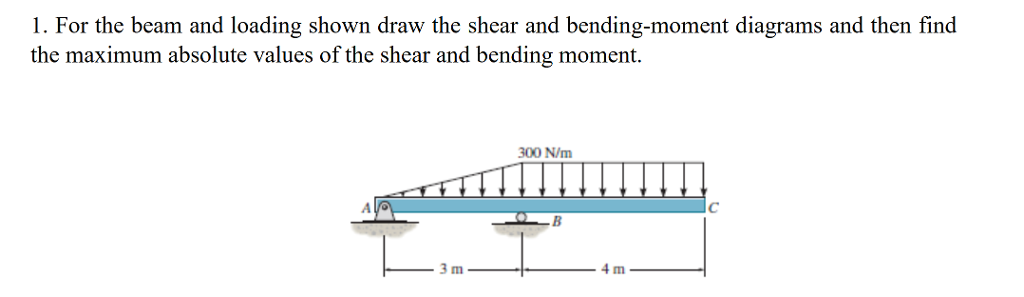For the beam and loading shown draw the shear and bending moment diagrams and then find the maximum absolute values of the shear and bending moment. 1. For the beam and loading shown draw the shear and bending-moment diagrams and then find the maximum absolute values of the shear and bending moment. 300 N/m 4 m

• ### PROBLEM 6 120 Nm 50 N с D For the beam and loading shown, (a) draw...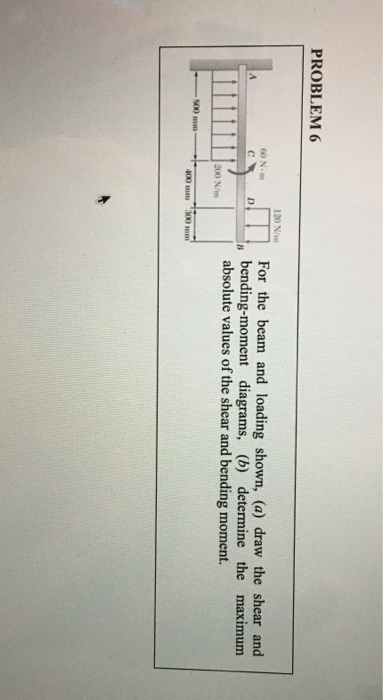PROBLEM 6 120 Nm 50 N с D For the beam and loading shown, (a) draw the shear and bending-moment diagrams, (b) determine the maximum absolute values of the shear and bending moment. 200 N/m 400 mm 300 mm

• ### PROBLEM 6 120 Nm 50 N с D For the beam and loading shown, (a) draw...PROBLEM 6 120 Nm 50 N с D For the beam and loading shown, (a) draw the shear and bending-moment diagrams, (b) determine the maximum absolute values of the shear and bending moment. 200 N/m 400 mm 300 mm

• ### PROBLEM 6 120 N/m 60 N.m D For the beam and loading shown, (a) draw the...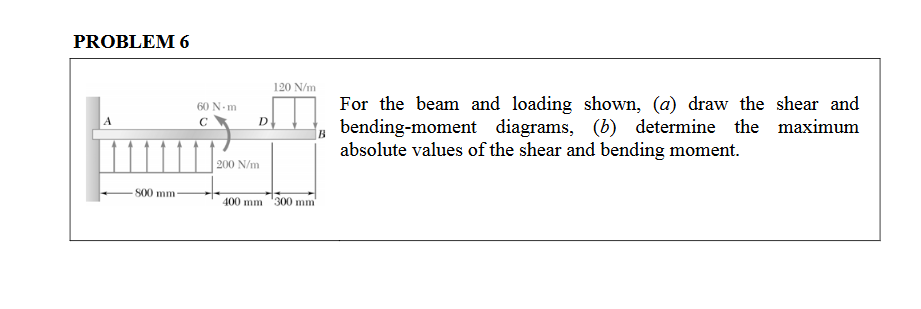PROBLEM 6 120 N/m 60 N.m D For the beam and loading shown, (a) draw the shear and bending-moment diagrams, (b) determine the maximum absolute values of the shear and bending moment. 200 N/m 500 mm 400 mm 300 mm

• ### For the beam and loading shown below: a. Draw the shear and bending moment diagrams. b....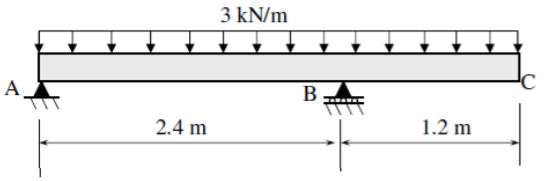For the beam and loading shown below: a. Draw the shear and bending moment diagrams. b. Determine the maximum absolute values of the shear and bending moment. 3 kN/m С B 2.4 m 1.2 m

• ### 1. For the beam and loading shown, (a) draw the shear and bending-moment diagrams, (b) determine...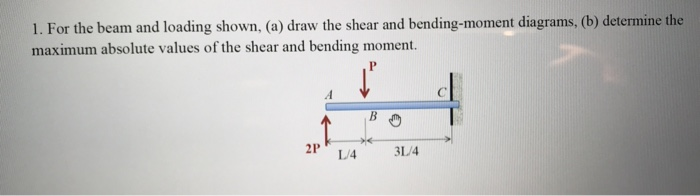1. For the beam and loading shown, (a) draw the shear and bending-moment diagrams, (b) determine the maximum absolute values of the shear and bending moment. L/4 3L4

• ### Problem 1A,B: Draw the shear and bending moment diagrams for the beam and loading shown, and...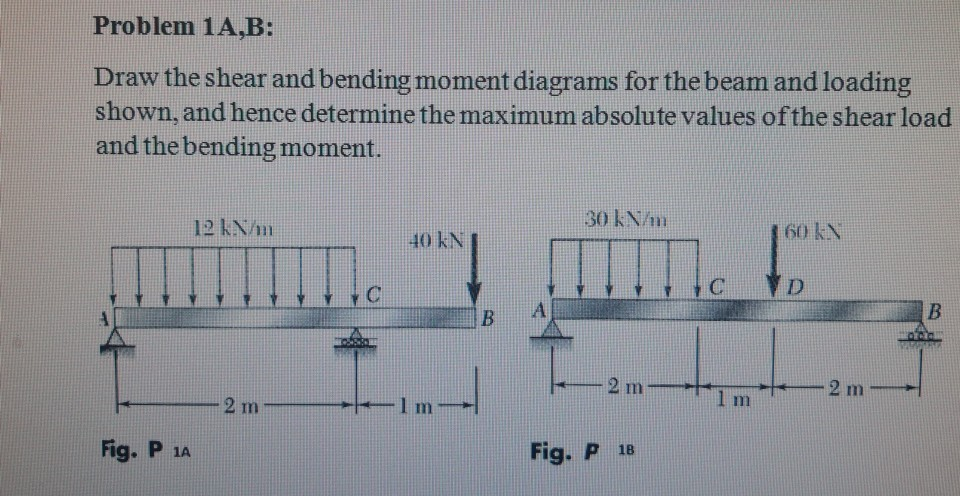Problem 1A,B: Draw the shear and bending moment diagrams for the beam and loading shown, and hence determine the maximum absolute values of the shear load and the bending moment. 30 kN/m 12 km 160 kN D 2 m 2 m 1 m 2 mm Fig. P 12 Fig. P 18

• ### 4. For the beam and loading shown, draw the shear force and bending moment diagrams and...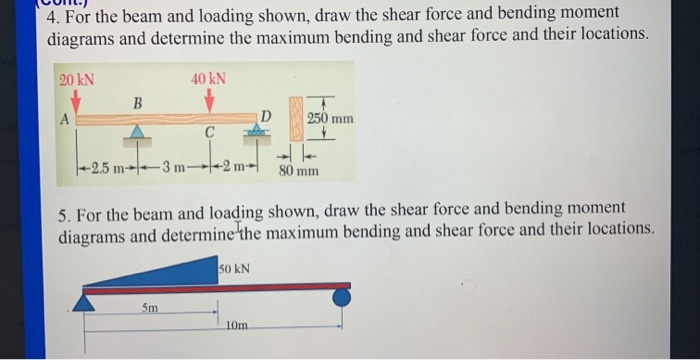4. For the beam and loading shown, draw the shear force and bending moment diagrams and determine the maximum bending and shear force and their locations. 20 KN 40 KN B D 250 mm |--2.5 m- 3m-4-2 m 80 mm 5. For the beam and loading shown, draw the shear force and bending moment diagrams and determine the maximum bending and shear force and their locations. 50 KN

• ### 7.69 and 7.70For the beam and loading shown, (a) draw the shear and bending-moment diagrams, (b)...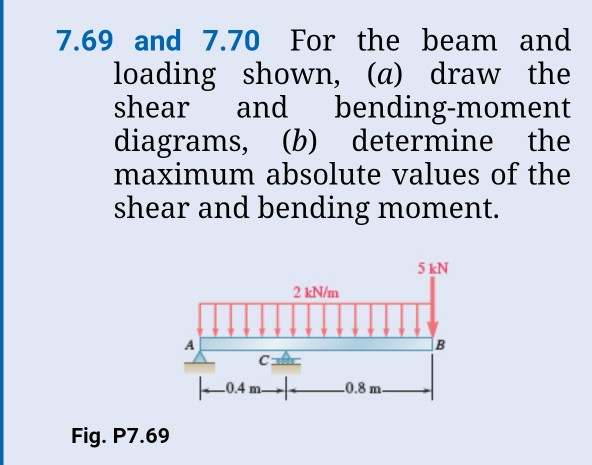7.69 and 7.70For the beam and loading shown, (a) draw the shear and bending-moment diagrams, (b) determine the maximum absolute values of the shear and bending moment. 5 kN 2 kN/m 0.8 m 0.4 Fig. P7.69